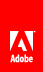# Using Point objects

Flash Player 9 and later, Adobe AIR 1.0 and later

A Point object defines a Cartesian pair of coordinates. It represents location in a two-dimensional coordinate system, where x represents the horizontal axis and y represents the vertical axis.

To define a Point object, you set its x and y properties, as follows:

```import flash.geom.*;
var pt1:Point = new Point(10, 20); // x == 10; y == 20
var pt2:Point = new Point();
pt2.x = 10;
pt2.y = 20;```

## Finding the distance between two points

You can use the distance() method of the Point class to find the distance between two points in a coordinate space. For example, the following code finds the distance between the registration points of two display objects, circle1 and circle2 , in the same display object container:

```import flash.geom.*;
var pt1:Point = new Point(circle1.x, circle1.y);
var pt2:Point = new Point(circle2.x, circle2.y);
var distance:Number = Point.distance(pt1, pt2);```

## Translating coordinate spaces

If two display objects are in different display object containers, they can be in different coordinate spaces. You can use the localToGlobal() method of the DisplayObject class to translate the coordinates to the same (global) coordinate space, that of the Stage. For example, the following code finds the distance between the registration points of two display objects, circle1 and circle2 , in the different display object containers:

```import flash.geom.*;
var pt1:Point = new Point(circle1.x, circle1.y);
pt1 = circle1.localToGlobal(pt1);
var pt2:Point = new Point(circle2.x, circle2.y);
pt2 = circle2.localToGlobal(pt2);
var distance:Number = Point.distance(pt1, pt2);```

Similarly, to find the distance of the registration point of a display object named target from a specific point on the Stage, use the localToGlobal() method of the DisplayObject class:

```import flash.geom.*;
var stageCenter:Point = new Point();
stageCenter.x = this.stage.stageWidth / 2;
stageCenter.y = this.stage.stageHeight / 2;
var targetCenter:Point = new Point(target.x, target.y);
targetCenter = target.localToGlobal(targetCenter);
var distance:Number = Point.distance(stageCenter, targetCenter);```

## Moving a display object by a specified angle and distance

You can use the polar() method of the Point class to move a display object a specific distance by a specific angle. For example, the following code moves the myDisplayObject object 100 pixels by 60°:

```import flash.geom.*;
var distance:Number = 100;
var angle:Number = 2 * Math.PI * (90 / 360);
var translatePoint:Point = Point.polar(distance, angle);
myDisplayObject.x += translatePoint.x;
myDisplayObject.y += translatePoint.y;```

## Other uses of the Point class

You can use Point objects with the following methods and properties:

Class

Methods or properties

Description

DisplayObjectContainer

`areInaccessibleObjectsUnderPoint()getObjectsUnderPoint()`

Used to return a list of objects under a point in a display object container.

BitmapData

hitTest()

Used to define the pixel in the BitmapData object as well as the point that you are checking for a hit.

BitmapData

applyFilter()

copyChannel()

merge()

paletteMap()

pixelDissolve()

threshold()

Used to define the positions of rectangles that define the operations.

Matrix

deltaTransformPoint()

transformPoint()

Used to define points for which you want to apply a transformation.

Rectangle

bottomRight

size

topLeft

Used to define these properties.// Ethnio survey code removed